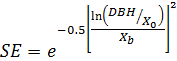SORTIE-ND
Software for spatially-explicit simulation of forest dynamics

# Competition Mortality

Competition mortality is a growth-based mortality behavior. It uses the results of the NCI growth behavior.

Trees killed by this behavior will have a mortality reason code of "natural".

### Parameters for this behavior

Parameter nameDescription
Competition Mortality Shape Parameter (Z)Determines the shape of the mortality function.
Competition Mortality Maximum Parameter (max)The maximum relative increment of growth subject to mortality.

### How it works

NCI growth in SORTIE is calculated in the following way:

Growth = Max Growth * Size Effect * Shading Effect * Crowding Effect * Damage Effect

Max Growth is the maximum diameter growth the tree can attain, in cm/yr, entered in the NCI Maximum Potential Growth, cm/yr parameter. Size Effect, Shading Effect, Crowding Effect, and Damage Effect are all factors which act to reduce the maximum growth rate and will vary depending on the conditions a tree is in. Each of these effects is a value between 0 and 1.

In the Competition mortality behavior, the following measure is used as predictor variable for probability of mortality:

Relative increment = Growth / PG

The relative increment is the ratio between the growth for an individual tree and the maximum growth possible for that tree. The Growth is the tree's growth for the previous timestep. PG is calculated as follows:

PG = Max Growth * SE

where Max Growth is the NCI growth parameter NCI Maximum Potential Growth, cm/yr, and SE is the Size Effect. Size Effect is calculated as follows:where:

• DBH is of the target tree, in cm
• X0 is the NCI Size Effect Mode, in cm (X0) NCI growth parameter
• Xb is the NCI Size Effect Variance, in cm (Xb) NCI growth parameter

Once the relative increment for an individual tree has been calculated, the probability of mortality for that individual is calculated in the following way:

Prob = Z relative increment / max

where:

• Prob is the probability of mortality
• Z is the Competition Mortality Shape Parameter (Z) parameter
• max is the Competition Mortality Maximum Parameter (max) parameter, which indicates the maximum relative increment subject to mortality

All trees with a relative increment greater than or equal to max will live.

### How to apply it

This behavior can be applied to saplings and adults of any species. It cannot be applied to seedlings. Any tree species/type combination to which it is applied must also have NCI growth. This behavior can only be applied with a one year timestep.